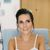# QlikView App Dev

Discussion Board for collaboration related to QlikView App Development.

Announcements
cancel
Showing results for
Did you mean:Creator III

## set analysis problem

whats wrong in this expression:

Sum({<Month={'<=month(today())'}>}Target_Product)

i want the sum of Target_Product till the end of the month

tnx.

1 Solution

Accepted SolutionsSpecialist II

Try this sorry for previous one

=Sum({<Month={"=(Month>(Month(today())))"}>}Target_Product)

12 RepliesMVP

Try like:

Sum({<Month={"<=\$(=month(today()))"}>}Target_Product)Creator III
Author

tnx

but its not working... =/MVP

I guess the month data format could be the issue. Could you post your sample app?Creator III
Author

this expression works:

=Sum({<Month={"=(month(today()))"}>}Target_Product)

gives only the target of the current monthNot applicable

When using month() function, it will return Feb, Oct according to your parameters. = is OK, <= is not.

You should do num(month(today()))

When you perform date comparison, you should use numeric.

FabriceMVP

Hi,

Use proper function name inside ""

Like use =Month(Today())

Great dreamer's dreams never fulfilled, they are always transcended.MVP

This has given a new finding:

Fabrice Aunez , comparison works (because with Month() the output is actually a dual), but with a different style:

=Sum({<Month={"=Month>Month(Today())"}>}Amt)    // Set analysis example

=Sum(If(Month>Month(today()),Amt))              // If

So, adi arnon, this : Sum({<Month={"=Month<=Month(Today())"}>}Target_Product)        , would work for you.

PFANot applicable

Tresesco,

I partially agree.

These two syntaxes work:

sum ({<Month ={"<= \$(=num(month(today())))"} >} Amount)

sum({<Month ={"=Month<Month(today()) "} >} Amount)

This syntax does not work (I do not get any result): The only difference with the first syntax is the num() function that is not used.

sum({<Month ={"<= \$(=month(today()))"} >} Amount)

Month is dual: it has been constructed using the Month() function.

In set analysis, I think it is better to use numeric for date comparisons. But (you are right) that is not the single way.

FabriceCreator III
Author

i tried this:

=Sum({<Month={"<=\$(month(today()))"}>}Target_Product)

its giving me the target of the whole year. nit just till this month